#### You may also like### Tri.'s

How many triangles can you make on the 3 by 3 pegboard?### Cutting Corners

Can you make the most extraordinary, the most amazing, the most unusual patterns/designs from these triangles which are made in a special way?### Bracelets

Investigate the different shaped bracelets you could make from 18 different spherical beads. How do they compare if you use 24 beads?

# Triangle Transformation

##### Age 7 to 14Challenge Level
Deana from KIS International School in Thailand/ Taiwan wrote:

First of all draw a right triangle, then cut it in four pieces that are right triangle too. Then it doesn't matter which one you put first because it is the same size, and for me, I made the length of the right triangle 6cm and 8.5cm which that means it is an isosceles triangle. I made 4 tiny triangles and the length is 4.25 and 3 cm.

Letizia from Bangkok Patana in Thailand sent in the following:

To complete this challenging task, I drew an isosceles triangle (with a ruler) on my squared paper to make it easier. Then I cut my triangle out, so I could do the next step. After that, I got my isosceles triangle and cut it in half and then I attached the two pieces back together so now
instead of being an isosceles triangle it was a rectangle.

Here are pictures to show step by step how I completed this process.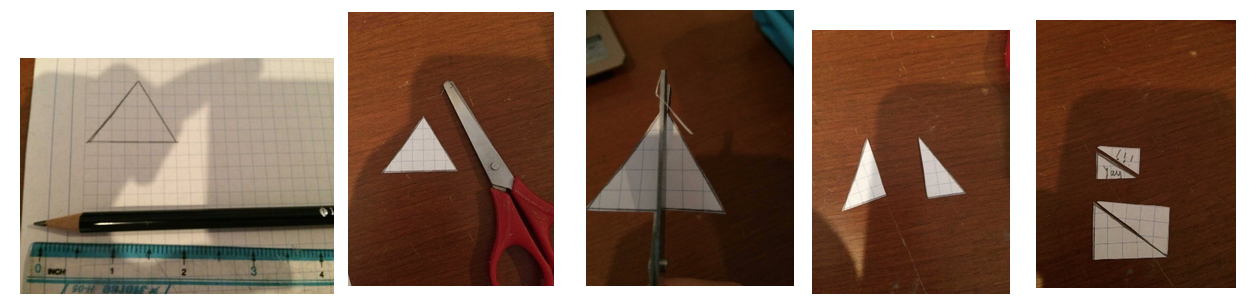These pupils from  Broughton Anglican College in Australia sent in their ideas

To complete this activity what I did was I found a triangle and ruled then cut it and I then equally halved it then put two sides together to make a rectangle.  Remember a rectangle is technically a four-sided shape!

Ebony
The answer is yes because if you get a triangle and cut it in half to make two triangles and put them together it will make a rectangle because two triangles always make a rectangle.

Austin
Cut your triangle into a quadrilateral triangle then cut it right through the middle and then put the to sides back together.

Sam
Cut out a rectangle then gut the rectangle from corner to corner diagonally done.

Laiba from Doha College in Qatar sent in the following excellent work:

There are many different ways to cut and rearrange the pieces of a rectangle into a square. The least amount of pieces that you would need to cut your rectangle into would be two.

If you start with a rectangle with dimensions of the ratio 4:1, you can cut it in half vertically and put one half on top of the other to make a square. This is the only way that you can make a square out of a rectangle by cutting it into only two pieces.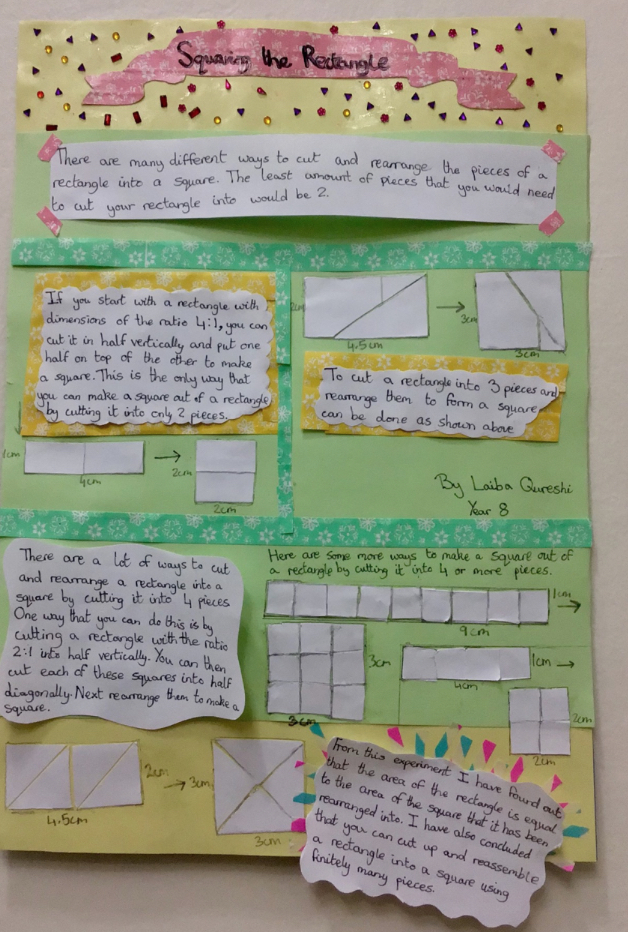see this clearer here.jpg

EDSR from the  Priestlands School in the United Kingdom sent in the following:

These are the triangles I am working with. The lines inside the triangles are the places you should cut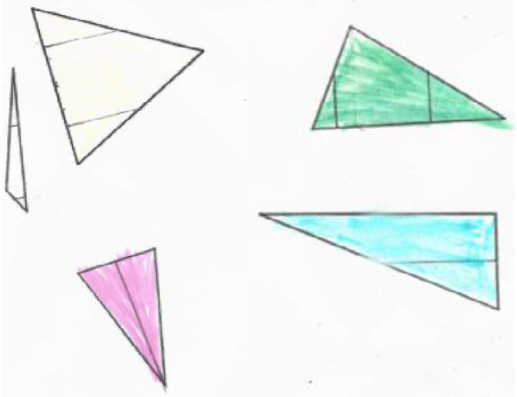These are the triangles rearranged; as you can see these rectangles used to be triangles: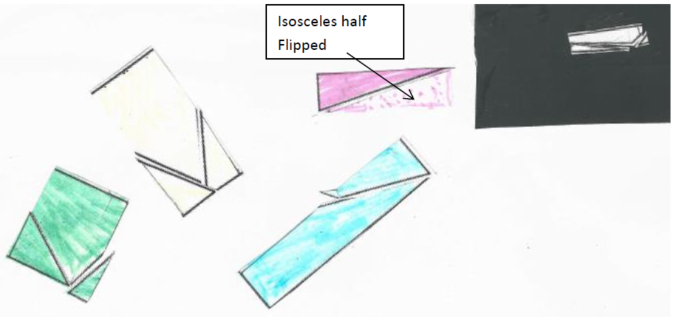Answer to Question: Out of all the Triangles I have worked with, you can always make a rectangle by cutting them fewer than four times.

We had four solutions from Norwich Lower School. Firstly, here's what Poppy and George did:

We found all different triangles the equilateral, the isosceles, the scalene and the right angle. We started with an isosceles and used four small triangles from the big triangles to make a rectangle. The scalene took us quite a lot of time as we tried equal pieces but then realised we needed irregular pieces. We think it is possible as we think we got them all. The rest were quite similar.

From Tom and Isaac: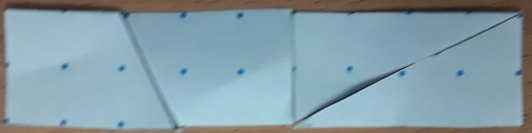We started with an isosceles triangle then we cut it into four pieces then we managed to make it into a rectangle.

From Else and William: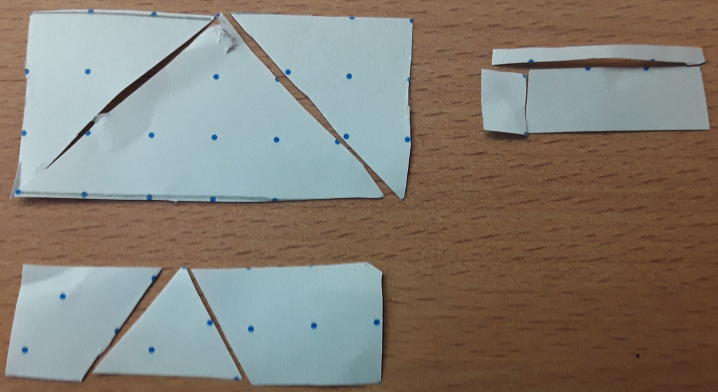First, we cut out a big equilateral triangle and drew a rectangle, cut the rectangle out as well as cutting the rectangle in smaller pieces then put it together.

Finally from William and India:
We started with an isosceles triangle and then we split it into two pieces. We then adjusted it and made it into a small rectangle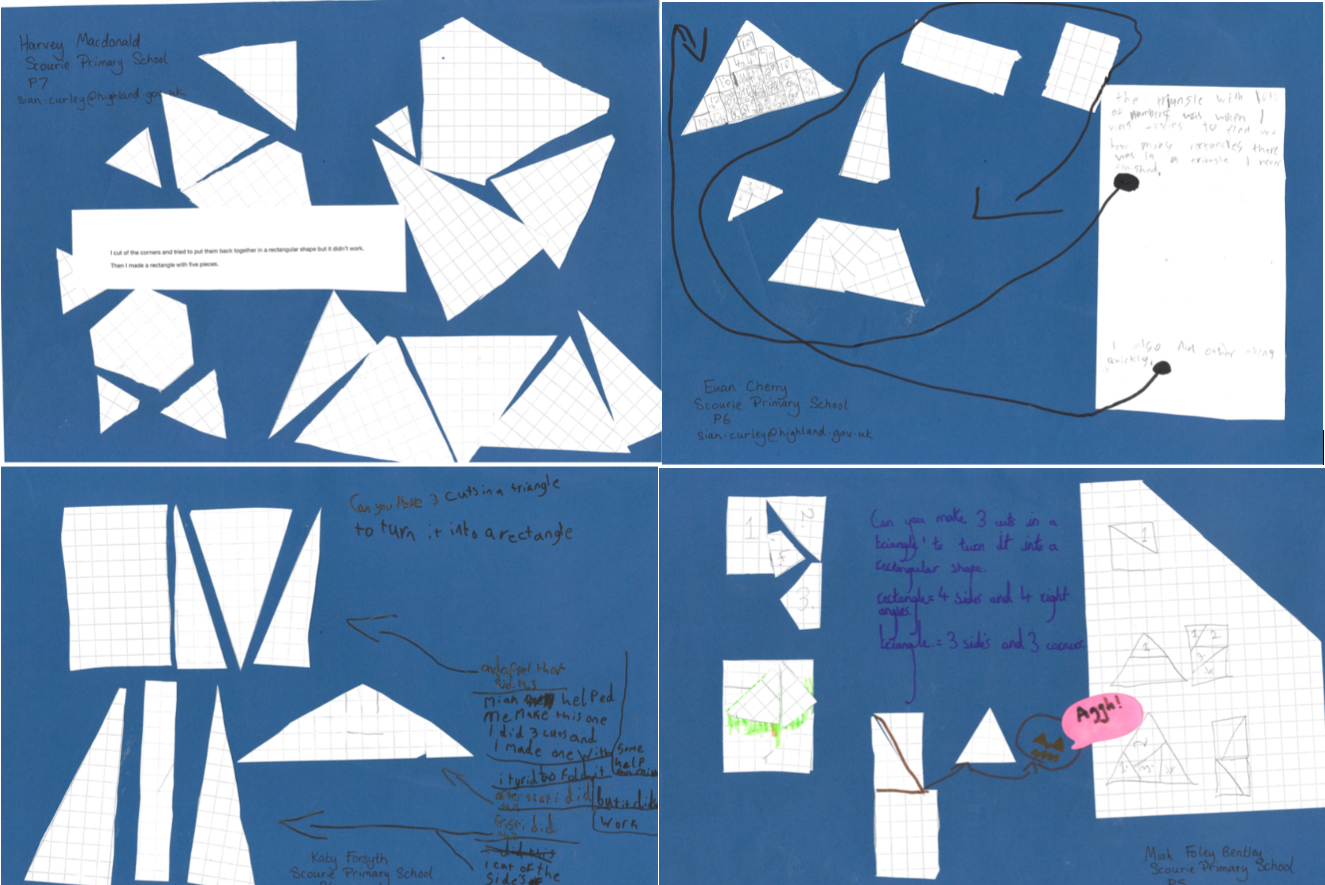From a small school in the very north of Scotland - Scourie Primary - we had these four pictures.  You can see then enlarged here.jpg.
Their teachers said that although they
didn't have time to find a method that would work for every triangle, they decided to split up the task so each experimented with a different idea.

Thank you everyone for sharing your solutions.